##### PostgreSQL array_fill() Function

PostgreSQL offers many array functions that are used to return some information about the array or manipulate the array in different ways. Array_fill() function is one of these functions that are used to manipulate the array. The basic functionality this function provides is that it fills the array with a specified element. The element and the dimension of the array are passed in the function as arguments.

In this post, we will examine how the array_fill() function works.

## How Does the PostgreSQL array_fill() Function work?

The array_fill() function fills the array with an element that is specified in the function as an argument. The dimension of the array is also specified as an argument. The basic syntax of the function is given below:

`array_fill(Element , Dimension[, lower_bound]) ;`

In the above syntax:

● The first Argument is the element that we want to fill in the array.

● The second argument specifies the dimension of the array for example, if Array is specified, it means that the array is one-dimensional and the length of the array is 4. If Array[3,4] is specified, this represents that the array is 2-dimensional, and the first dimension of the array is 3 and the second one is 4.

● The final argument is entirely optional. This determines the array's starting index.

The return values of this function have a data type of ARRAY. The function returns an array of a dimension specified and having an element that is also specified as an argument.

Let’s move towards the example to get more clarity on the concept.

Example

Let's first consider a simple example of a one-dimensional array. The query is given below:

`SELECT array_fill(3, ARRAY);`

In the above query:

● The first argument is the element 3. It means the array will be filled with the element 3.

● The second element depicts the dimension of the array. Here in this case it is Array, which means that the array will be one-dimensional and will have the length 4.

So the output of the given query will be: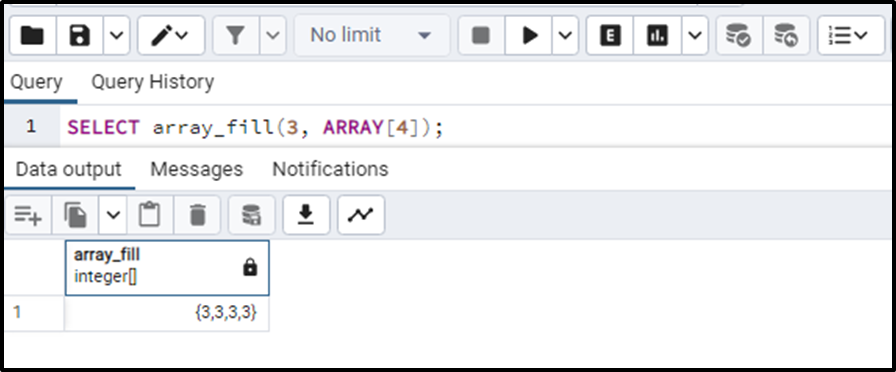We can also specify the lower bound for this function which is completely optional, but let’s see how it works when it is specified:

`SELECT array_fill(3, ARRAY ,ARRAY);`

In the above query, the lower bound “ARRAY” is specified which means that the array will be starting from the index position of 2. The output will specify the starting and ending index of the array in case the lower bound is specified in the query. The output will be as follows: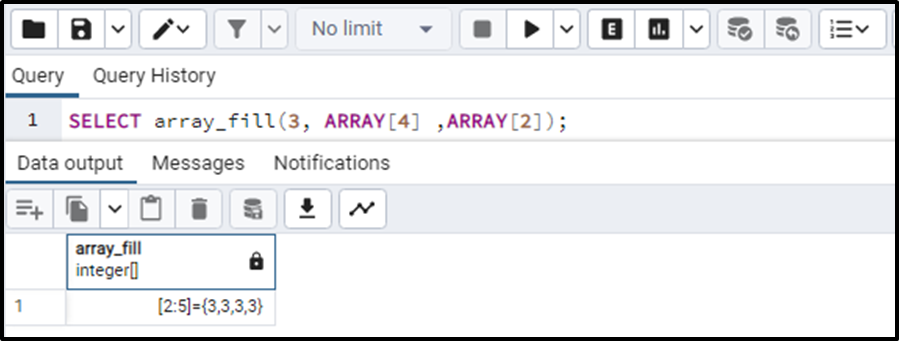The resulting array contains element 3 and has a length of 4, while the array is starting with an index of 2 to 5.

Now consider another example of a multi-dimensional array. The query for this case is given below:

`SELECT array_fill(3, ARRAY[4,2]);`

In this query, the element will be 3. The second argument depicts that the array is 2-dimensional. The first dimension is 4 and the second dimension is 2. Let’s see what the output of the query looks like: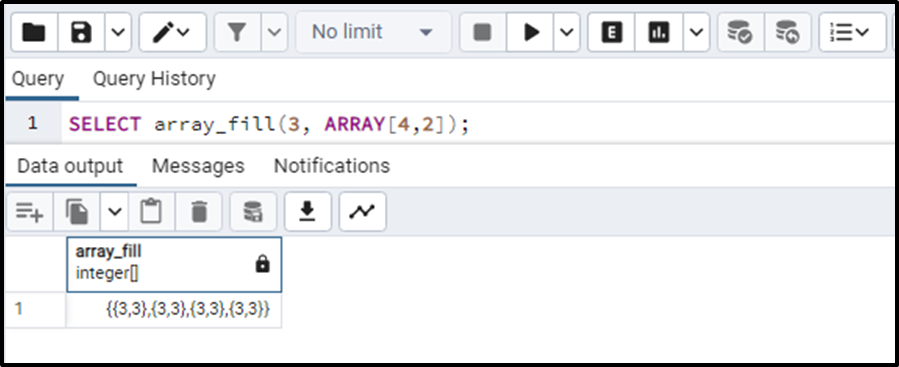In the above output, it is clearly illustrated that the resulting array is 2-dimensional having a first dimension of 4 and a second dimension of 2.

Another multi-dimensional array is as follows:

`SELECT array_fill(3, ARRAY[4,2,2]);`

The resulting array is 3 dimensional, having a first dimension of 4, a second dimension of 2, and a third dimension of 2 as well. The output is illustrated below: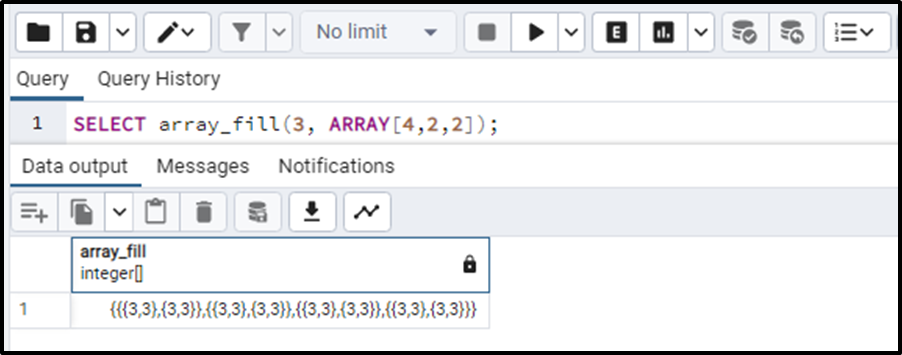### Input Type Error

If we want to fill our array with an element “abc”, we will write the query as:

`SELECT array_fill('abc', ARRAY[4,2]);`

By executing this query you will see that the output will be an error saying; ERROR: could not determine polymorphic type because input has type unknown.

This means that we have not specified the data type of the element. To fix this error we need to specify the data type in the query like this:

`SELECT array_fill('abc'::TEXT, ARRAY[4,2]);`

This will resolve the issue and give us our required output like this: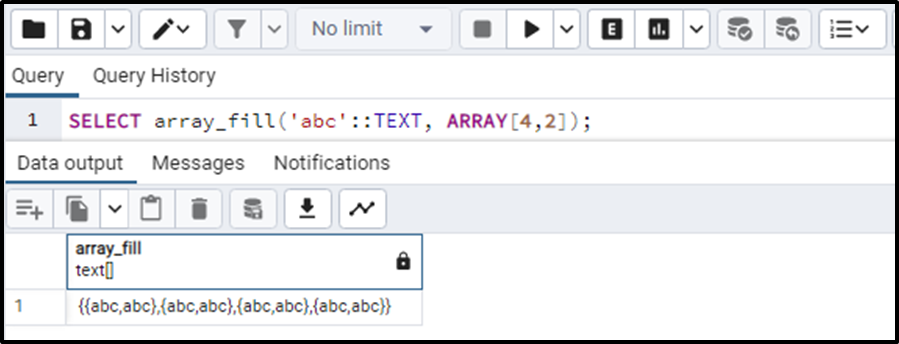This is how the array_fill() function works in PostgreSQL.

## Conclusion

The array_fill() is a function that fills an array with a specified argument. The dimensions of that array are also specified in that function as an argument. The return values of this function have a data type of ARRAY. This article demonstrated how to utilize the array_fill() function in PostgreSQL.